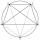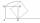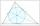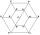High school + geometry construction problems - examples

1. Z9–I–1In all nine fields of given shape to be filled natural numbers so that: • each of the numbers 2, 4, 6 and 8 is used at least once, • four of the inner square boxes containing the products of the numbers of adjacent cells of the outer square, • in the cir
2. Square gridSquare grid consists of a square with sides of length 1 cm. Draw in it at least three different patterns such that each had a content of 6 cm2 and circumference 12 cm and that their sides is in square grid.
3. Circle tangentIt is given to a circle with the center S and radius 3.5 cm. Distance from the center to line p is 6 cm. Construct a circle tangent n which is perpendicular to the line p.
4. 10 piecesHow to divide the circle into 10 parts (geometrically)?
5. Mrak - cloudIt is given segment AB of length 12 cm, where one side of the square MRAK laid on it. MRAK's side length 2 cm shown. MRAK gradually flips along the line segment AB the point R leaves a paper trail. Draw the whole track of point R until square can do the.
6. Inscribed circleWrite the equation of a incircle of the triangle KLM if K [2,1], L [6,4], M [6,1].
7. Square ABCDConstruct a square ABCD with cente S [3,2] and the side a = 4 cm. Point A lies on the x-axis. Construct square image in the displacement given by oriented segment SS'; S` [-1 - 4].
8. Hexagon = 8 partsDivide the regular hexagon into eight equal parts.
9. Resultant forceCalculate mathematically and graphically the resultant of a three forces with a common centre if: F1 = 50 kN α1 = 30° F2 = 40 kN α2 = 45° F3 = 40 kN α3 = 25°
10. ConstructConstruct a triangle ABC inscribed circle has a radius r = 2 cm, the angle alpha = 50 degrees = 8 cm. Make a sketch, analysis, construction and description.

We apologize, but in this category are not a lot of examples.
Do you have an interesting mathematical example that you can't solve it? Enter it, and we can try to solve it.

To this e-mail address, we will reply solution; solved examples are also published here. Please enter e-mail correctly and check whether you don't have a full mailbox.